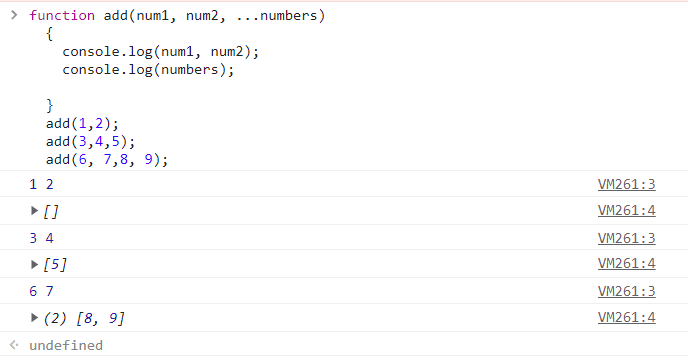# Rest Parameter in JavaScript | Example Program

Rest parameter is an important feature provided by JavaScript. It allows us to send an arbitrary (indefinite) number of arguments to a function in the form of an array.

It is suitable in scenarios where the number of input parameters to a function is indefinite. The concept of rest parameter was introduced in ES6 (sixth version of ECMAScript).

We define a rest parameter in JavaScript using rest operator (…) in the parameter list. The rest parameter signifies the parameter can accept any number of arguments.

The general syntax to use a rest parameter in the parameter list is as follows:

```function functionName(p1, p2, ....parameters) {
// code to be executed.
}
```

Putting a rest operator (…) before the last formal parameter (parameters) shows that the parameter is rest parameter. The rest parameter can only be one and it will be the last one in the list of parameters.

## JavaScript Rest Parameters Example

Let us consider a simple function add(), which adds two numbers. Suppose we need to add three numbers, then we will create a new function with three parameters.

Similarly, to add four numbers, we will have to create again a new function with four parameters.

What will you do if we need a function where the number of parameters can vary? For instance, we need a function that can accept any number of arguments and compute the results.

We can achieve it using the rest parameters. The rest parameters have a prefix of three dots that allow to accept an indefinite number of arguments in a function. They represent these arguments as an array.

Let’s try to understand the concept of rest parameters with the help of an example.As you can observe in the above screenshot, we have created a function which accepts two primary arguments num1 and num2.

In the function definition shown in the first line of above figure, after writing parameters num1 and num2, we have defined a rest parameter denoted by a prefix of three dots (…) and variable by a name numbers.

This rest parameter numbers can store many parameters as an array. We do not need to create multiple new functions for different number of parameters. We can send any number of arguments and the function will handle all of them.

As you can see in the above screenshot, we have sent two arguments, as in, add(1, 2), the rest parameter shows an empty array.

Next, we have sent three arguments, as in, add(3, 4, 5), we will have third argument 4 in the array form.

Finally, we have sent four arguments, as in, add(6, 7, 8, 9), we will have two argument values 8 and 9 in the array form.

## JavaScript Rest Parameter Restriction

Rest parameter has two restrictions that are as:

1. The first restriction is that there can be only one rest parameter and the rest parameter must be the last in the function parameter list. For example, the below code will not work:

```// Syntax error: Can't have a named parameter after rest parameters.
{
// do something.
}
```

2. The second restriction is that we cannot use rest parameters in an object literal setter. This means that the following code would also cause a syntax error:

```let object = {
// Syntax error: Can't use rest parameter in the setter.
set name(...value) {
// do something.
}
};```

## Rest Parameters Example Program

Consider the following example programs based on rest parameters in JavaScript.

Program code 1:

```<html>
<title>JavaScript Rest Parameters Example Program</title>
<script>
function myFunc(name1, name2, ...namen)
{
document.write("First name: " +name1, "<br>");
document.write("Second name: " +name2, "<br>");
for(let i = 0; i < namen.length; i++)
{
document.write("Other name: " +namen[i], "<br>");
}
}
myFunc("John", "Tripti", "Priya", "Ivaan", "Deep" );
</script>
<body>
</body>
</html>
```
```Output:
First name: John
Second name: Tripti
Other name: Priya
Other name: Ivaan
Other name: Deep
```

2. Let’s take an example program in which we will calculate the sum of numbers using rest parameters and display the result on the browser.

Program code 2:

```<html>
<title>JavaScript Rest Parameters Example Program</title>
<script>
function myFunc(num1, num2,...numn)
{
var sum = num1 + num2;
for(var i = 0; i < numn.length; i++)
{
sum = sum + numn[i];
}
document.write("Sum of numbers = " +sum);
}
myFunc(25, 30, 10, 5, 15, 20 );
</script>
<body>
</body>
</html>
```
```Output:
Sum of numbers = 105
```

In this example, we have declared a function with rest parameter and invoked it. The first two parameters are regular parameters and the rest parameters are rest parameters in the parameter list.

JavaScript automatically takes all the arguments we have passed to the function and clubs it into an array. The function then iterates the array and performs sum operations on all the input elements supplied.

Program code 3:

```<html>
<title>JavaScript Rest Parameter Example</title>
<script>
function myFunc(...numn)
{
var sum = 0;
for(var i = 0; i < numn.length; i++)
{
sum = sum + numn[i];
}
document.write("Sum of numbers = " +sum);
}
myFunc(25, 30, 10, 5, 15, 20 );
</script>
</html>
```
```Output:
Sum of numbers = 105
```

In this example program, all the arguments that we have passed to the parameter list of the function are rest parameters.

JavaScript interpreter automatically accepts all the arguments passed to the parameter list and clubs it into an array. Then, the function iterates through array and performs sum operations on all input elements supplied.

In this tutorial, you leaned rest parameter in JavaScript with various example programs. Hope that you will have understood the basic concept of rest parameters.